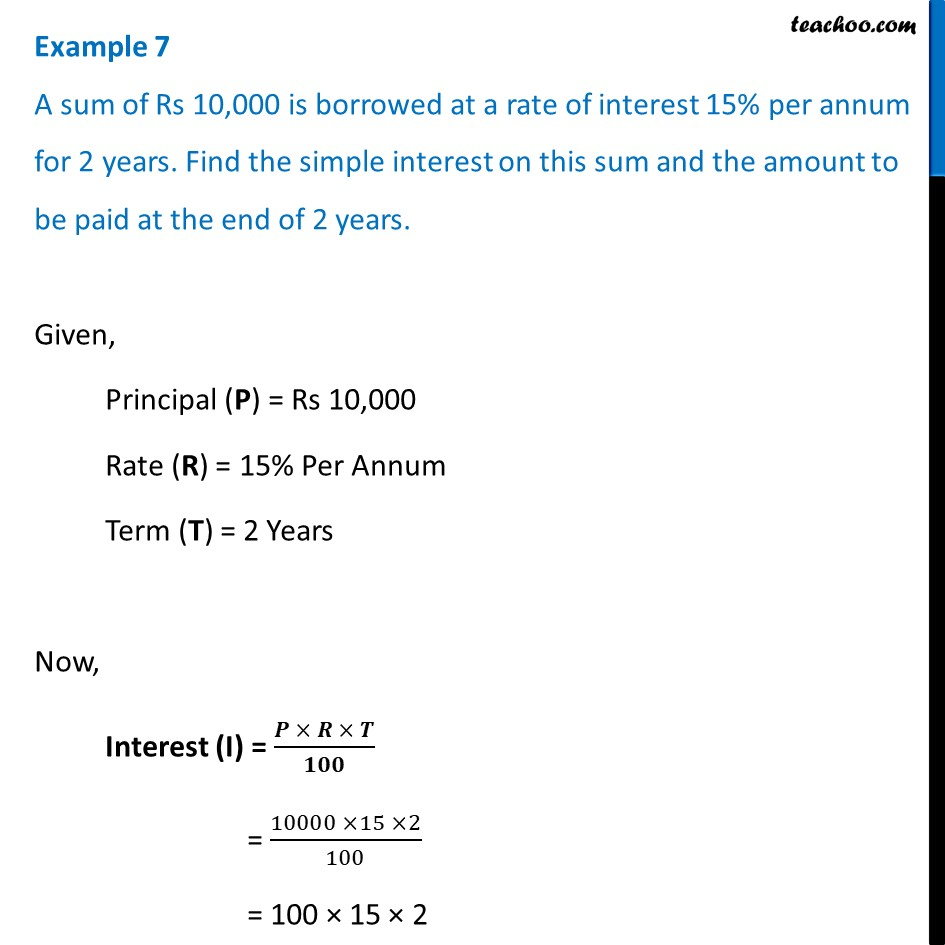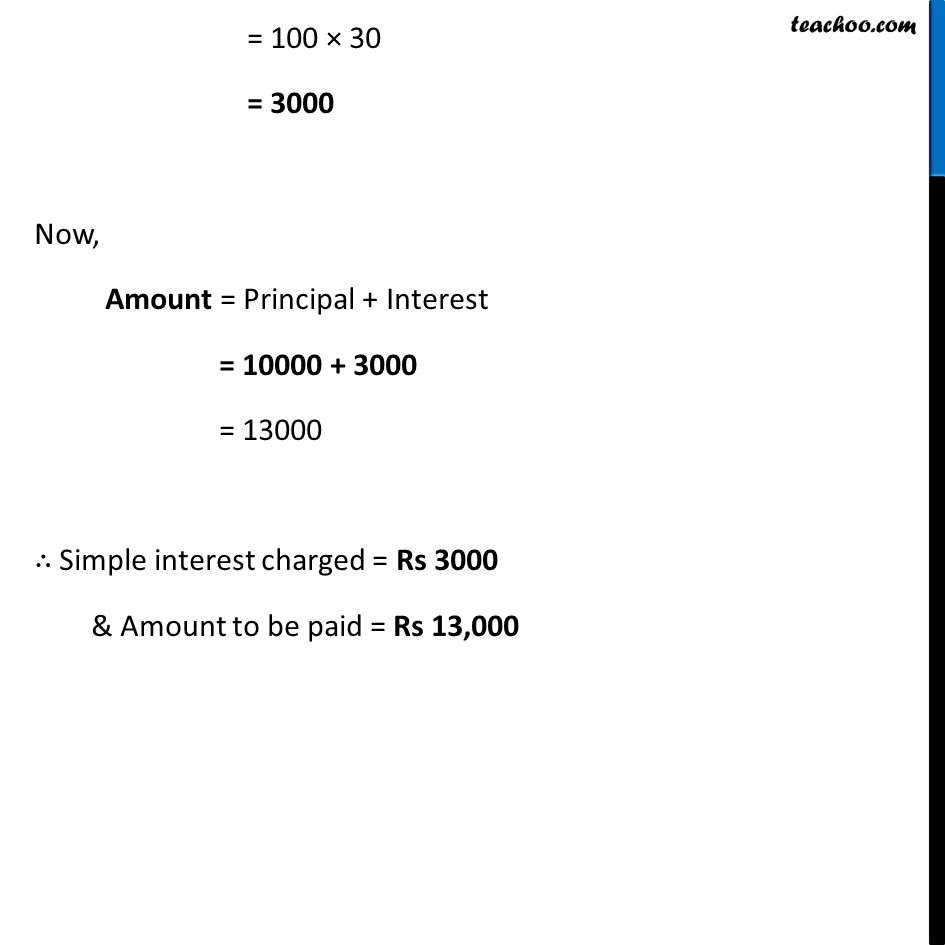Examples

Chapter 7 Class 8 Comparing Quantities
Serial order wiseLearn in your speed, with individual attention - Teachoo Maths 1-on-1 Class

### Transcript

Example 7 A sum of Rs 10,000 is borrowed at a rate of interest 15% per annum for 2 years. Find the simple interest on this sum and the amount to be paid at the end of 2 years.Given, Principal (P) = Rs 10,000 Rate (R) = 15% Per Annum Term (T) = 2 Years Now, Interest (I) = (𝑷 × 𝑹 × 𝑻)/𝟏𝟎𝟎 = (10000 ×15 ×2)/100 = 100 × 15 × 2 = 100 × 30 = 3000 Now, Amount = Principal + Interest = 10000 + 3000 = 13000 ∴ Simple interest charged = Rs 3000 & Amount to be paid = Rs 13,000